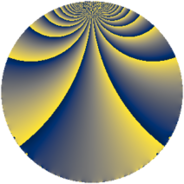# Properties

 Label 784.4.bgLevel $784$ Weight $4$ Character orbit 784.bg Rep. character $\chi_{784}(65,\cdot)$ Character field $\Q(\zeta_{21})$ Dimension $996$ Sturm bound $448$

# Related objects

## Defining parameters

 Level: $$N$$ $$=$$ $$784 = 2^{4} \cdot 7^{2}$$ Weight: $$k$$ $$=$$ $$4$$ Character orbit: $$[\chi]$$ $$=$$ 784.bg (of order $$21$$ and degree $$12$$) Character conductor: $$\operatorname{cond}(\chi)$$ $$=$$ $$49$$ Character field: $$\Q(\zeta_{21})$$ Sturm bound: $$448$$

## Dimensions

The following table gives the dimensions of various subspaces of $$M_{4}(784, [\chi])$$.

Total New Old
Modular forms 4104 1020 3084
Cusp forms 3960 996 2964
Eisenstein series 144 24 120

## Trace form

 $$996q + 19q^{3} - 13q^{5} - 6q^{7} + 716q^{9} + O(q^{10})$$ $$996q + 19q^{3} - 13q^{5} - 6q^{7} + 716q^{9} + 13q^{11} - 10q^{13} + 64q^{15} - 13q^{17} - 588q^{19} - 107q^{21} - 29q^{23} + 2046q^{25} - 368q^{27} - 150q^{29} + 1002q^{31} - 79q^{33} + 371q^{35} - 13q^{37} + 1666q^{39} - 306q^{41} - 326q^{43} - 456q^{45} - 559q^{47} + 476q^{49} + 417q^{51} + 183q^{53} + 72q^{55} + 293q^{57} + 1419q^{59} + 87q^{61} + 5096q^{63} - 28q^{65} - 78q^{67} + 504q^{69} + 290q^{71} + 1079q^{73} - 592q^{75} + 393q^{77} - 876q^{79} + 3625q^{81} - 3834q^{83} - 260q^{85} - 2336q^{87} + 631q^{89} + 3074q^{91} + 66q^{93} - 696q^{95} + 2304q^{97} + 2796q^{99} + O(q^{100})$$

## Decomposition of $$S_{4}^{\mathrm{new}}(784, [\chi])$$ into newform subspaces

The newforms in this space have not yet been added to the LMFDB.

## Decomposition of $$S_{4}^{\mathrm{old}}(784, [\chi])$$ into lower level spaces

$$S_{4}^{\mathrm{old}}(784, [\chi]) \cong$$ $$S_{4}^{\mathrm{new}}(49, [\chi])$$$$^{\oplus 5}$$$$\oplus$$$$S_{4}^{\mathrm{new}}(98, [\chi])$$$$^{\oplus 4}$$$$\oplus$$$$S_{4}^{\mathrm{new}}(196, [\chi])$$$$^{\oplus 3}$$$$\oplus$$$$S_{4}^{\mathrm{new}}(392, [\chi])$$$$^{\oplus 2}$$